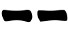## Sunday, 3 July 2016

This method was devised by Jason A. Wolcott and recorded in the I Ching methods page on the Lucky Mojo site.
It uses two dices: one with 20 faces (1d20) and one with eight faces (1d8) which are very easy to find being commonly used for bard games.
It proceeds as follows:
1. Roll the dice;
2. Draw the line:
• If the 20 sided die shows an even number (2,4,...,20), draw;
• If the 8 sided die shows 1, it's a moving line
• If the 20 sided die shows an odd number (1,3,...,19), draw;
•  If the 8 sided die shows 6, 7 or 8, it's a moving line
3. Repeat steps 1-2 other five times drawing the hexagram from the bottom to the top

#### Probabilities

The 20 sided die gives the nature of the line (yin or yang) with probability 1/2. The process is designed to give the same probability of the Method of 16 one:
Prob(6) = 1/2 * 1/8 = 1/16
Prob(8) = 1/2 * 7/8 = 7/16
Prob(7) = 1/2 * 5/8 = 5/16
Prob(9) = 1/2 * 3/8 = 3/16
Prob(yin) = Prob(yang) = 1/2

#### Variations

Since the the 20 sided die is only used for even/odd probability it can be replaced by any die with an even number of faces.